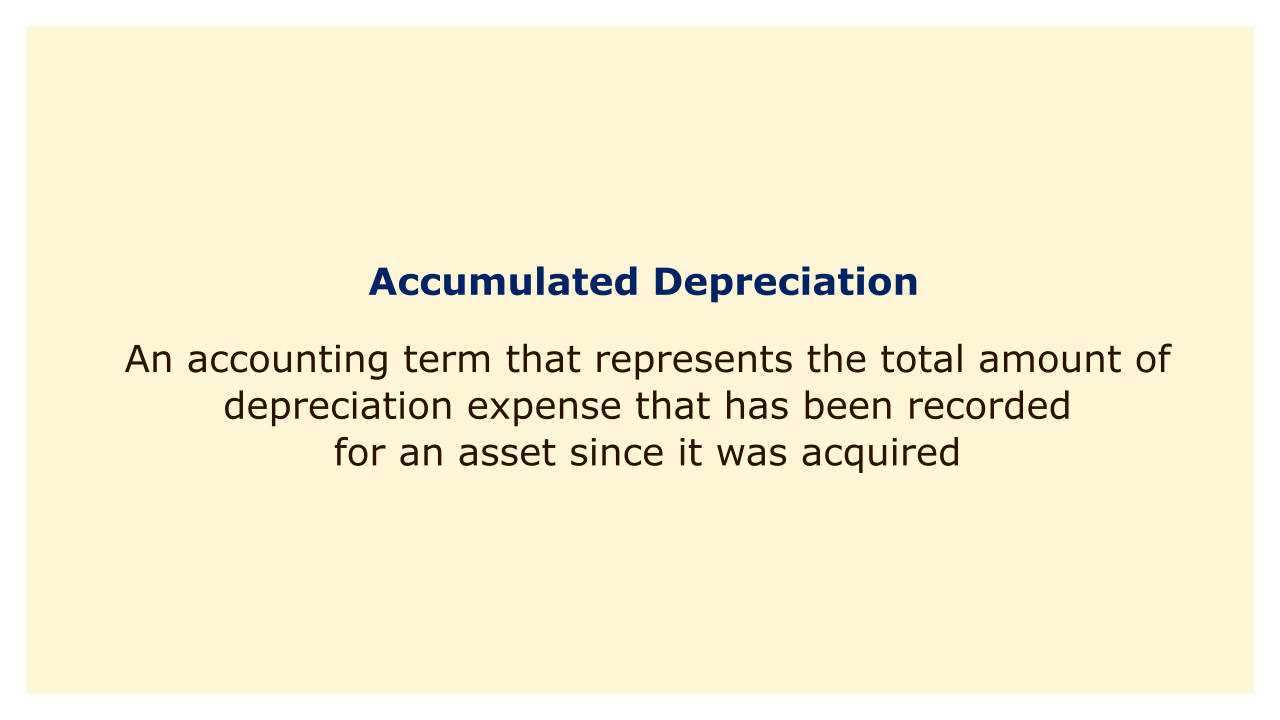# Accumulated DepreciationImage: Moneybestpal.com

### In accounting, the term "accumulated depreciation" refers to the total amount of depreciation expense that has been tallied for an asset after its acquisition. Being a contra-asset account with a credit balance, accumulated depreciation lowers an asset's carrying value on the balance sheet.

Allocating a long-term asset's cost over the course of its useful life is called depreciation. A depreciation charge lowers a company's taxable income as well as its net income. There are several ways to figure the depreciation expense, including straight-line, declining balance, units of production, and the sum of the years' digits.

Accumulated depreciation is calculated by adding up all the depreciation expense that has been recorded for an asset over time. As an illustration, if a business spends \$10,000 on a machine and uses the straight-line technique to depreciate it over ten years with no salvage value, the yearly depreciation expense will be \$1,000. The machine will have a carrying value of \$5,000 and \$5,000 worth of depreciation after five years.

The book value of an asset can also be calculated using accumulated depreciation. The difference between an asset's acquisition cost and total depreciation is its book value. When assessing an asset's performance and profitability, book value is the accounting term for the asset's net worth. To evaluate if an asset is overvalued or undervalued, book value can be contrasted with market value.

Accumulated depreciation can also be used to calculate the gain or loss on the disposal of an asset. The difference between the money obtained and the asset's book value must be recorded by the company as a gain or loss on disposal when an asset is sold or retired. For instance, if a corporation sells a machine for \$4,000 while recognizing a loss of \$1,000 on disposal, the machine's book value is \$5,000.

The idea of accumulated depreciation is crucial in accounting because it illustrates how much of an asset's original cost has been depleted over time. Companies can present their assets at their net worth on the balance sheet and match their revenues and costs in accordance with the matching principle thanks to accumulated depreciation.
Tags